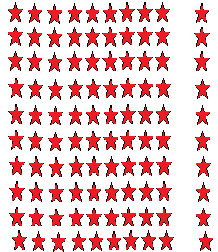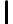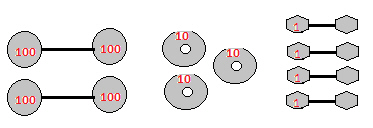﻿ 2NBTst1PostName:    2nd Grade Numbers in Operations in Base Ten- Understand place value, Standard 1, Post Test 1 Understand that the three digits of a three-digit number represent amounts of hundreds, tens, and ones; e.g., 706 equals 7 hundreds, 0 tens, and 6 ones.

Multiple Choice
Identify the choice that best completes the statement or answers the question.

1.What is the sum of all the stars?
 a. 10 c. 100 b. 90 d. 80

2.

In the number 999, what is the value of the second 9?
 a. 9 c. 900 b. 90 d. 18

3.

What is the total of the 10’s in the array?a. 100 c. 10 b. 1000 d. 20

4.

In the number 437, what is the place value of the7.
 a. 437 c. 37 b. 7 d. 1

5.

In the number 822, what is the value of the 8?
 a. 12 c. 800 b. 14 d. 80

Numeric Response

6.

THE NEXT QUESTIONS ARE ALL ANSWERED WITH JUST A NUMBER.
In the number below, which number is in the tens place?
919

7.

In the number below, which number is in the ones place?
315

8.

In the number below, which number is in the hundreds place?
146

9.

In the number below, which number is in the hundreds place?
5683

10.

In the number 100, how many tens are there?

11.

In the number 900, how many hundreds are there?

12.

In the number 999, how many ones are there?

13.

In the number 89164, which number holds the hundreds place value?

14.

What number does this diagram represent?

100’s                   10’s                          1’s
•••••15.

What number does this diagram represent? (Count each red number)16.

Sandy lives on a farm.  She has six barns that each holds one-hundred chickens.  She has two barns that each hold ten cows and one barn that holds three horses.  What is the sum of all the animals on Sandy’s farm?